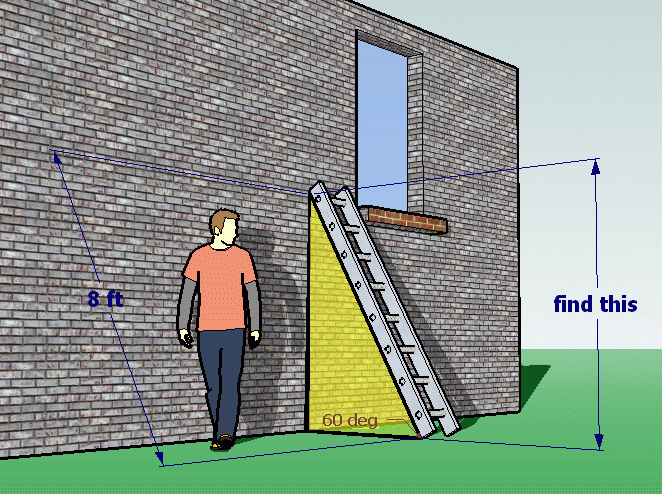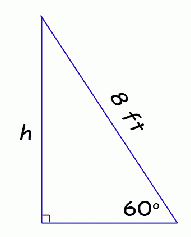SEARCH HOMEMath Central Quandaries & QueriesQuestion from madi, a student: A ladder 8 ft long resting on a house makes a 60 degree angle with the ground. how far up the house does it reach?I sketched the question below.Can you see the yellow right-angled triangle? That's what you use to find the answer for the question. Here's a simpler geometric sketch. We'll call the length of the side we want to find h.There is a trig function called sine which will let you find the value of h.

In any right triangle, the length of the leg opposite a particular angle is equal to the length of the hypotenuse times the sine of the angle.

So

h = (8 ft) sin (60°)

Hope this helps,
Stephen La Rocque.Math Central is supported by the University of Regina and The Pacific Institute for the Mathematical Sciences.